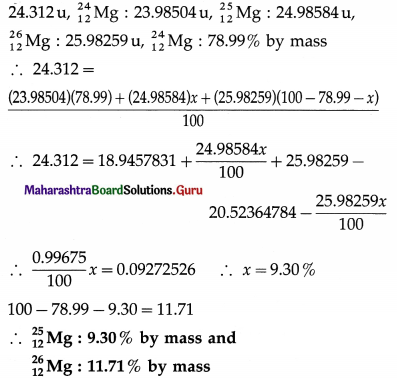# Maharashtra Board Class 12 Physics Solutions Chapter 15 Structure of Atoms and Nuclei

Balbharti Maharashtra State Board 12th Physics Textbook Solutions Chapter 15 Structure of Atoms and Nuclei Textbook Exercise Questions and Answers.

## Maharashtra State Board 12th Physics Solutions Chapter 15 Structure of Atoms and Nuclei

In solving problems, use me = 0.00055 u = 0.5110 MeV/c2, mp = 1.00728 u, mn = 1.00866u, mH = 1.007825 u, u = 931.5 MeV, e = 1.602 × 10-19 C, h = 6.626 × 10-34 Js, ε0 = 8.854 × 10-12 SI units and me = 9.109 × 10-31 kg.

1. Choose the correct option.

i) In which of the following systems will the radius of the first orbit of the electron be the smallest?
(A) hydrogen
(B) singly ionized helium
(C) deuteron
(D) tritium
(D) tritium

ii) The radius of the 4th orbit of the electron will be smaller than its 8th orbit by a factor of
(A) 2
(B) 4
(C) 8
(D) 16
(B) 4iii) In the spectrum of hydrogen atom which transition will yield longest wavelength?
(A) n = 2 to n = 1
(B) n = 5 to n = 4
(C) n = 7 to n = 6
(D) n = 8 to n = 7
(D) n = 8 to n = 7

iv) Which of the following properties of a nucleus does not depend on its mass number?
(B) mass
(C) volume
(D) density
(D) density

v) If the number of nuclei in a radioactive sample at a given time is N, what will be the number at the end of two half-lives?
(A) $$\frac{N}{2}$$
(B) $$\frac{N}{4}$$
(C) $$\frac{3N}{4}$$
(D) $$\frac{N}{8}$$
(B) $$\frac{N}{4}$$

i) State the postulates of Bohr’s atomic model.
The postulates of Bohr’s atomic model (for the hydrogen atom) :

1. The electron revolves with a constant speed in acircular orbit around the nucleus. The necessary centripetal force is the Coulomb force of attraction of the positive nuclear charge on the negatively charged electron.
2. The electron can revolve without radiating energy only in certain orbits, called allowed or stable orbits, in which the angular momentum of the electron is equal to an integral multiple of h/2π, where h is Planck’s constant.
3. Energy is radiated by the electron only when it jumps from one of its orbits to another orbit having lower energy. The energy of the quantum of elec-tromagnetic radiation, i.e., the photon, emitted is equal to the energy difference of the two states.

ii) State the difficulties faced by Rutherford’s atomic model.
(1) According to Rutherford, the electrons revolve in circular orbits around the atomic nucleus. The circular motion is an accelerated motion. According to the classical electromagnetic theory, an accelerated charge continuously radiates energy. Therefore, an electron during its orbital motion, should go on radiating energy. Due to the loss of energy, the radius of its orbit should go on decreasing. Therefore, the electron should move along a spiral path and finally fall into the nucleus in a very short time, of the order of 10-16 s in the case of a hydrogen atom. Thus, the atom should be unstable. We exist because atoms are stable.

(2) If the electron moves along such a spiral path, the radius of its orbit would continuously decrease. As a result, the speed and frequency of revolution of the electron would go on increasing. The electron, therefore, would emit radiation of continuously changing frequency, and hence give rise to a con-tinuous spectrum. However, atomic spectrum is a line spectrum.iii) What are alpha, beta and gamma decays?
(a) A radioactive transformation in which an α-particle is emitted is called α-decay.
In an α-decay, the atomic number of the nucleus decreases by 2 and the mass number decreases by 4.
Example : $${ }_{92}^{238} \mathrm{U} \rightarrow{ }_{90}^{234} \mathrm{Th}+{ }_{2}^{4} \alpha$$
Q = [mu – mTh – mα]c2

(b) A radioactive transformation in which a β-particle is emitted is called β-decay.
In a β-decay, the atomic number of the nucleus increases by 1 and the mass number remains unchanged.
Example : $${ }_{90}^{234} \mathrm{Th} \rightarrow{ }_{91}^{234} \mathrm{~Pa}+{ }_{-1}^{0} e+\bar{v}_{\mathrm{e}}$$
where $$\bar{v}_{\mathrm{e}}$$ is the neutrino emitted to conserve the momentum, energy and spin.
Q = [mu – mTh – mα]c2
In a β+-decay, the atomic number of the nucleus decreases by 1 and the mass number remains unchanged.
Example : \begin{aligned} &30 \\ &15 \end{aligned} P \rightarrow{ }_{14}^{30} \mathrm{Si}+{ }_{+1}^{0} e+v_{\mathrm{e}}
where ve is the neutrino emitted to conserve the momentum, energy and spin.
Q = [mP – mSi – me]c2
[Note : The term fi particle refers to the electron (or positron) emitted by a nucleus.]

A given nucleus does not emit α and β-particles simultaneously. However, on emission of α or β-particles, most nuclei are left in an excited state. A nucleus in an excited state emits a γ-ray photon in a transition to the lower energy state. Hence, α and β-particle emissions are often accompanied by γ-rays.

iv) Define excitation energy, binding energy and ionization energy of an electron in an atom.
(1) Excitation energy of an electron in an atom : The energy required to transfer an electron from the ground state to an excited state (a state of higher energy) is called the excitation energy of the electron in that state.

(2) Binding energy of an electron in an atom is defined as the minimum energy that should be provided to an orbital electron to remove it from the atom such that its total energy is zero.

(3) Ionization energy of an electron in an atom is defined as the minimum energy required to remove the least strongly bound electron from a neutral atom such that its total energy is zero.

v) Show that the frequency of the first line in Lyman series is equal to the difference between the limiting frequencies of Lyman and Balmer series.
For the first line in the Lyman series,
$$\frac{1}{\lambda_{\mathrm{L} 1}}=R\left(\frac{1}{1^{2}}-\frac{1}{2^{2}}\right)=R\left(1-\frac{1}{4}\right)=\frac{3 R}{4}$$
∴ vL1 = $$\frac{c}{\lambda_{\mathrm{L} 1}}=\frac{3 R_{c}}{4}$$, where v denotes the frequency,
c the speed of light in free space and R the Rydberg constant.
For the limit of the Lyman series,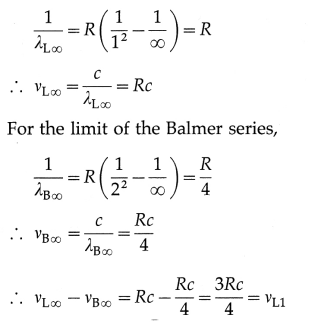Hence, the result.

Question 3.
State the postulates of Bohr’s atomic model and derive the expression for the energy of an electron in the atom.
The postulates of Bohr’s atomic model (for the hydrogen atom) :
(1) The electron revolves with a constant speed in acircular orbit around the nucleus. The necessary centripetal force is the Coulomb force of attraction of the positive nuclear charge on the negatively charged electron.
(2) The electron can revolve without radiating energy only in certain orbits, called allowed or stable orbits, in which the angular momentum of the electron is equal to an integral multiple of h/2π, where h is Planck’s constant.
(3) Energy is radiated by the electron only when it jumps from one of its orbits to another orbit having lower energy. The energy of the quantum of elec-tromagnetic radiation, i.e., the photon, emitted is equal to the energy difference of the two states.

Consider the electron revolving in the nth orbit around the nucleus of an atom with the atomic number Z. Let m and e be the mass and the charge of the electron, r the radius of the orbit and v the linear speed of the electron.

According to Bohr’s first postulate, centripetal force on the electron = electrostatic force of attraction exerted on the electron by the nucleus
∴ $$\frac{m v^{2}}{r}=\frac{1}{4 \pi \varepsilon_{0}} \cdot \frac{Z e^{2}}{r^{2}}$$ ……………. (1)
where ε0 is the permittivity of free space.
∴ Kinetic energy (KE) of the electron
= $$\frac{1}{2} m v^{2}=\frac{Z e^{2}}{8 \pi \varepsilon_{0} r}$$ ………….. (2)
The electric potential due to the nucleus of charge +Ze at a point at a distance r from it is
V = $$\frac{1}{4 \pi \varepsilon_{0}} \cdot \frac{\mathrm{Ze}}{r}$$
∴ Potential energy (PE) of the electron
= charge on the electron × electric potential
= – e × $$\frac{1}{4 \pi \varepsilon_{0}} \frac{Z e}{r}=-\frac{Z e^{2}}{4 \pi \varepsilon_{0} r}$$ …………….. (3)
Hence, the total energy of the electron in the nth orbit is
E = KE + PE = $$\frac{-Z e^{2}}{4 \pi \varepsilon_{0} r}+\frac{Z e^{2}}{8 \pi \varepsilon_{0} r}$$
∴ E = $$-\frac{Z e^{2}}{8 \pi \varepsilon_{0} r}$$ ………….. (4)
This shows that the total energy of the electron in the nth orbit of the atom is inversely proportional to the radius of the orbit as Z, ε0 and e are constants. The radius of the nth orbit of the electron is
r = $$\frac{\varepsilon_{0} h^{2} n^{2}}{\pi m Z e^{2}}$$ …………….. (5)
where h is Planck’s constant.
From Eqs. (4) and (5), we get,
En = $$-\frac{Z e^{2}}{8 \pi \varepsilon_{0}}\left(\frac{\pi m Z e^{2}}{\varepsilon_{0} h^{2} n^{2}}\right)=-\frac{m Z^{2} e^{4}}{8 \varepsilon_{0}^{2} h^{2} n^{2}}$$ ……………… (6)
This gives the expression for the energy of the electron in the nth Bohr orbit. The minus sign in the expression shows that the electron is bound to the nucleus by the electrostatic force of attraction.
As m, Z, e, ε0 and h are constant, we get
En ∝ $$\frac{1}{n^{2}}$$
i.e., the energy of the electron in a stationary energy state is discrete and is inversely proportional to the square of the principal quantum number.
[ Note : Energy levels are most conveniently expressed in electronvolt. Hence, substituting the values of m, e, £0 and h, and dividing by the conversion factor 1.6 × 10-19 J/eV,
En ≅ $$-\frac{13.6 Z^{2}}{n^{2}}$$ (in eV)
For hydrogen, Z = 1
∴ En ≅ $$-\frac{13.6}{n^{2}}$$ (in eV).

Question 4.
Starting from the formula for energy of an electron in the nth orbit of hydrogen atom, derive the formula for the wavelengths of Lyman and Balmer series spectral lines and determine the shortest wavelengths of lines in both these series.
According to Bohr’s third postulate for the model of the hydrogen atom, an atom radiates energy only when an electron jumps from a higher energy state to a lower energy state and the energy of the
quantum of electromagnetic radiation emitted in this process is equal to the energy difference between the two states of the electron. This emission of radiation gives rise to a spectral line.

The energy of the electron in a hydrogen atom,
when it is in an orbit with the principal quantum
number n, is
En = $$-\frac{m e^{4}}{8 \varepsilon_{0}^{2} h^{2} n^{2}}$$
where m = mass of electron, e = electronic charge, h = Planck’s constant and = permittivity of free space.

Let Em be the energy of the electron in a hydrogen atom when it is in an orbit with the principal quantum number m and E, its energy in an orbit with the principal quantum number n, n < m. Then
Em = $$-\frac{m e^{4}}{8 \varepsilon_{0}^{2} h^{2} m^{2}}$$ and En = $$-\frac{m e^{4}}{8 \varepsilon_{0}^{2} h^{2} m^{2}}$$
Therefore, the energy radiated when the electron jumps from the higher energy state to the lower energy state is
Em – En = $$\frac{-m e^{4}}{8 \varepsilon_{0}^{2} h^{2} m^{2}}-\left(-\frac{m e^{4}}{8 \varepsilon_{0}^{2} h^{2} n^{2}}\right)$$
= $$\frac{m e^{4}}{8 \varepsilon_{0}^{2} h^{2}}\left(\frac{1}{n^{2}}-\frac{1}{m^{2}}\right)$$
This energy is emitted in the form of a quantum of radiation (photon) with energy hv, where V is the frequency of the radiation.
∴ Em – En = hv
∴ v = $$\frac{E_{m}-E_{n}}{h}=\frac{m e^{4}}{8 \varepsilon_{0}^{2} h^{3}}\left(\frac{1}{n^{2}}-\frac{1}{m^{2}}\right)$$
The wavelength of the radiation is λ = $$\frac{c}{v^{\prime}}$$
where c is the speed of radiation in free space.
The wave number, $$\bar{v}=\frac{1}{\lambda}=\frac{v}{c}$$
$$\bar{v}=\frac{1}{\lambda}=\frac{m e^{4}}{8 \varepsilon_{0}^{2} h^{3} c}\left(\frac{1}{n^{2}}-\frac{1}{m^{2}}\right)=R\left(\frac{1}{n^{2}}-\frac{1}{m^{2}}\right)$$
where $$R\left(=\frac{m e^{4}}{8 \varepsilon_{0}^{2} h^{3} c}\right)$$ is a constant called the Ryd berg constant.

This expression gives the wave number of the radiation emitted and hence that of a line in hydrogen spectrum.

For the Lyman series, n = 1,m = 2, 3, 4, ………… ∞
∴ $$\frac{1}{\lambda_{\mathrm{L}}}=R\left(\frac{1}{1^{2}}-\frac{1}{m^{2}}\right)$$ and for the shortest wavelength line m this series, $$\frac{1}{\lambda_{\mathrm{Ls}}}=R\left(\frac{1}{1^{2}}\right)$$ as m = ∞.
For the Balmer series, n = 2, m = 3, 4, 5, … ∞.
∴ $$\frac{1}{\lambda_{\mathrm{B}}}=R\left(\frac{1}{4}-\frac{1}{m^{2}}\right)$$ and for the shortest wavelength line in this series, $$\frac{1}{\lambda_{\mathrm{Bs}}}=R\left(\frac{1}{4}\right)$$ as m = ∞
[Note: Johannes Rydberg (1854—1919), Swedish spectroscopist, studied atomic emission spectra and introduced the idea of wave number. The empirical formula $$\bar{v}=\frac{1}{\lambda}=R\left(\frac{1}{n^{2}}-\frac{1}{m^{2}}\right)$$ where m and n are simple integers, is due to Rydberg. When we consider the finite mass of the nucleus, we find that R varies slightly from element to element.]Question 5.
Determine the maximum angular speed of an electron moving in a stable orbit around the nucleus of hydrogen atom.
The radius of the ,ith Bohr orbit is
r = $$\frac{\varepsilon_{0} h^{2} n^{2}}{\pi m Z e^{2}}$$ ………….. (1)
and the linear speed of an electron in this orbit is
ν = $$\frac{Z e^{2}}{2 \varepsilon_{0} n h}$$ …………… (2)
where ε 0 permittivity of free space, h ≡ Planck’s constant, n ≡ principal quantum number, m ≡ electron mass, e electronic charge and Z ≡ atomic number of the atom.
Since angular speed ω = $$\frac{v}{r}$$, then from Eqs. (1) and (2), we get,
ω = $$\frac{v}{r}=\frac{Z e^{2}}{2 \varepsilon_{0} n h} \cdot \frac{\pi m Z e^{2}}{\varepsilon_{0} h^{2} n^{2}}=\frac{\pi m Z^{2} e^{4}}{2 \varepsilon_{0}^{2} h^{3} n^{3}}$$ ………………. (3)
which gives the required expression for the angular speed of an electron in the nth Bohr orbit.
From Eq. (3), the frequency of revolution of the electron,
f = $$\frac{\omega}{2 \pi}=\frac{1}{2 \pi} \times \frac{\pi m Z^{2} e^{4}}{2 \varepsilon_{0}^{2} h^{3} n^{3}}=\frac{m Z^{2} e^{4}}{4 \varepsilon_{0}^{2} h^{3} n^{3}}$$ …………….. (4)
as required.
[Note : From Eq. (4), the period of revolution of the electron, T = $$\frac{1}{f}=\frac{4 \varepsilon_{0}^{2} h^{3} n^{3}}{m Z e^{4}}$$. Hence, f ∝ $$\frac{1}{n^{3}}$$ and T ∝ n3].

Obtain the formula for ω and continue as follows :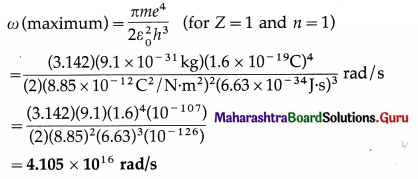This is required quantity.

Question 6.
Determine the series limit of Balmer, Paschen and Bracket series, given the limit for Lyman series is 912 Å.
Data : λL∞ = 912 Å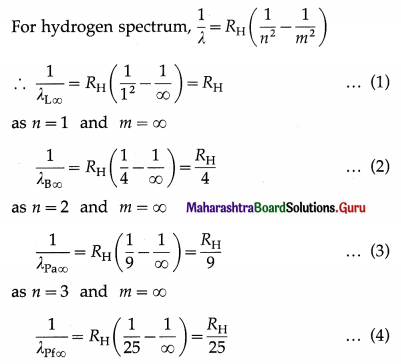as n = 5 and m = ∞
From Eqs. (1) and (2), we get,
$$\frac{\lambda_{\mathrm{Pa} \infty}}{\lambda_{\mathrm{L} \infty}}=\frac{R_{\mathrm{H}}}{R_{\mathrm{H}} / 9}$$ = 9
∴ λPa∞ = 9λL∞ = (9) (912) = 8202 Å
$$\frac{\lambda_{\mathrm{Pf} \infty}}{\lambda_{\mathrm{L} \infty}}=\frac{R_{\mathrm{H}}}{R_{\mathrm{H}} / 25}$$ = 25
∴ λPf∞ = 25λL∞ = (25) (912) = 22800 Å
This is the series limit of the pfund series.

Question 7.
Describe alpha, beta and gamma decays and write down the formulae for the energies generated in each of these decays.
(a) A radioactive transformation in which an α-particle is emitted is called α-decay.
In an α-decay, the atomic number of the nucleus decreases by 2 and the mass number decreases by 4.
Example : $${ }_{92}^{238} \mathrm{U} \rightarrow{ }_{90}^{234} \mathrm{Th}+{ }_{2}^{4} \alpha$$
Q = [mu – mTh – mα]c2

(b) A radioactive transformation in which a β-particle is emitted is called β-decay.
In a β-decay, the atomic number of the nucleus increases by 1 and the mass number remains unchanged.
Example : $${ }_{90}^{234} \mathrm{Th} \rightarrow{ }_{91}^{234} \mathrm{~Pa}+{ }_{-1}^{0} e+\bar{v}_{\mathrm{e}}$$
where $$\bar{v}_{\mathrm{e}}$$ is the neutrino emitted to conserve the momentum, energy and spin.
Q = [mu – mTh – mα]c2
In a β+-decay, the atomic number of the nucleus decreases by 1 and the mass number remains unchanged.
Example : \begin{aligned} &30 \\ &15 \end{aligned} P \rightarrow{ }_{14}^{30} \mathrm{Si}+{ }_{+1}^{0} e+v_{\mathrm{e}}
where ve is the neutrino emitted to conserve the momentum, energy and spin.
Q = [mP – mSi – me]c2
[Note : The term fi particle refers to the electron (or positron) emitted by a nucleus.]

A given nucleus does not emit α and β-particles simultaneously. However, on emission of α or β-particles, most nuclei are left in an excited state. A nucleus in an excited state emits a γ-ray photon in a transition to the lower energy state. Hence, α and β-particle emissions are often accompanied by γ-rays.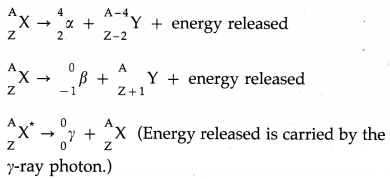Question 8.
Explain what are nuclear fission and fusion giving an example of each. Write down the formulae for energy generated in each of these processes.
Nuclear fission is a nuclear reaction in which a heavy nucleus of an atom, such as that of uranium, splits into two or more fragments of comparable size, either spontaneously or as a result of bombardment of a neutron on the nucleus (induced fission). It is followed by emission of two or three neutrons.
The mass of the original nucleus is more than the sum of the masses of the fragments. This mass difference is released as energy, which can be enormous as in the fission of 235U.
Nuclear fission was discovered by Lise Meitner, Otto Frisch, Otto Hahn and Fritz Strassmann in 1938.

The products of the fission of 235U by thermal neutrons are not unique. A variety of fission fragments are produced with mass number A ranging from about 72 to about 138, subject to the conservation of mass-energy, momentum, number of protons (Z) and number of neutrons (N). A few typical fission equations are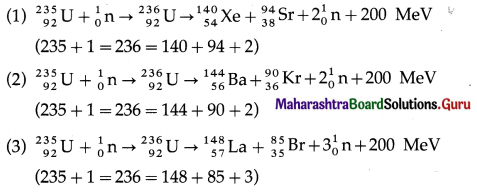A type of nuclear reaction in which lighter atomic nuclei (of low atomic number) fuse to form a heavier nucleus (of higher atomic number) with the’ release of enormous amount of energy is called nuclear fusion.

Very high temperatures, of about 107 K to 108 K, are required to carry out nuclear fusion. Hence, such a reaction is also called a thermonuclear reaction.

Example : The D-T reaction, being used in experimental fusion reactors, fuses a deuteron and a triton nuclei at temperatures of about 108 K.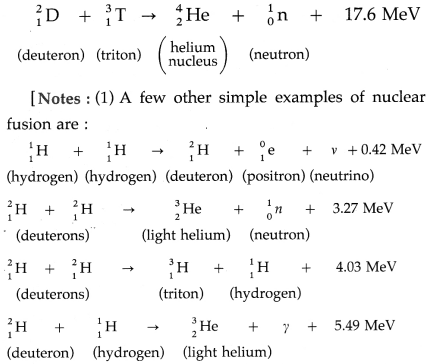(2) The value of the energy released in the fusion of two deuteron nuclei and the temperature at which the reaction occurs mentioned in the textbook are probably misprints.]Question 9.
Describe the principles of a nuclear reactor. What is the difference between a nuclear reactor and a nuclear bomb?
In a nuclear reactor fuel rods are used to provide a suitable fissionable material such as $$\begin{gathered} 236 \\ 92 \end{gathered}$$U. Control rods are used to start or stop the reactor. Moderators are used to slow down the fast neutrons ejected in a nuclear fission to the appropriate lower speeds. Material used as a coolant removes the energy released in the nuclear reaction by converting it into thermal energy for production of electricity.

In a nuclear reactor, a nuclear fission chain reaction is used in a controlled manner, while in a nuclear bomb, the nuclear fission chain reaction is not controlled, releasing tremendous energy in a very short time interval.
[Note : The first nuclear bomb (atomic bomb) was dropped on Hiroshima in Japan on 06 August 1945. The second bomb was dropped on Nagasaki in Japan on 9 August 1945.]

Question 10.
Calculate the binding energy of an alpha particle given its mass to be 4.00151 u.
Data : M = 4.00151 u, = 1.00728 u,
mn = 1.00866 u, 1 u = 931.5 MeV/c2
The binding energy of an alpha particle
(Zmp + Nn -M)c2
=(2mp + 2mn -M)c2
= [(2)(1.00728u) + 2(1.00866 u) – 4.00151 u]c2
= (2.01456 + 2.01732 – 4.00151)(931.5) MeV
= 28.289655 MeV
= 28.289655 × 106 eV × 1.602 × 10-19 J
= 4.532002731 × 10-12 J

Question 11.
An electron in hydrogen atom stays in its second orbit for 10-8 s. How many revolutions will it make around the nucleus in that time?
Data : z = 1, m = 9.1 × 10-31 kg, e = 1.6 × 10-19 C, ε0 = 8.85 × 10-12 C2 / N.m2, h = 6.63 × 10 -34 J.s, n = 2, t = 10-8 s
The periodic time of the electron in a hydrogen atom,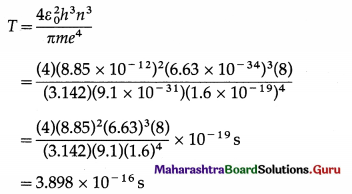Let N be the number of revolutions made by the electron in time t. Then, t = NT.
∴ N = $$\frac{t}{T}=\frac{10^{-8}}{3.898 \times 10^{-16}}$$ = 2.565 × 7

Question 12.
Determine the binding energy per nucleon of the americium isotope $$_{95}^{244} \mathrm{Am}$$ , given the mass of $${ }_{95}^{244} \mathrm{Am}$$ to be 244.06428 u.
Data : Z = 95, N = 244  – 95 = 149,
mp = 1.00728 u, mn = 1.00866 u,
M = 244.06428 u, 1 u = 931.5 MeV/c2
The binding energy per nucleon,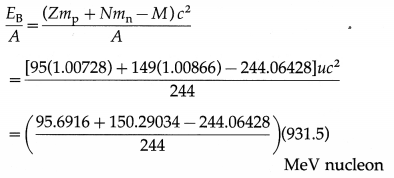= 7.3209 MeV/nucleon

Question 13.
Calculate the energy released in the nuclear reaction $${ }_{3}^{7} \mathrm{Li}$$ + p → 2α given mass of $${ }_{3}^{7} \mathrm{Li}$$ atom and of helium atom to be 7.016 u and 4.0026 u respectively.
Data: M1 ($${ }_{3}^{7} \mathrm{Li}$$ Li atom)= 7.016 u, M2 (He atom)
= 4.0026 u, mp = 1.00728 u, 1 u = 931.5 MeV/c2
∆M = M1 + mp – 2M2
= [7.016 + 1.00728 – 2(4.0026)]u
= 0.01808 u = (0.01808)(931.5) MeV/c2
= 16.84152 MeV/c2
Therefore, the energy released in the nuclear reaction = (∆M) c2 = 16.84152 MeV

Question 14.
Complete the following equations describing nuclear decays.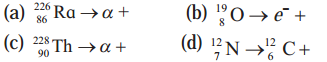(a) $${ }_{88}^{226} \mathrm{Ra} \rightarrow{ }_{2}^{4} \alpha+{ }_{86}^{222} \mathrm{Em}$$
Here, α particle is emitted and radon is formed.

(b) $${ }_{8}^{19} \mathrm{O} \rightarrow e^{-}+{ }_{9}^{19} \mathrm{~F}$$
Here, e ≡ $${ }_{-1}^{0} \beta$$ is emitted and fluorine is formed.

(c) $$\underset{90}{228} \mathrm{Th} \rightarrow{ }_{2}^{4} \alpha+{ }_{88}^{224} \mathrm{Ra}$$
Here, α particle is emitted and radium is formed.

(d) $${ }_{7}^{12} \mathrm{~N} \rightarrow{ }_{6}^{12} \mathrm{C}+{ }_{1}^{0} \beta$$
$${ }_{1}^{0} \beta$$ is e+ (positron)
Here, β+ is emItted and carbon is formed.Question 15.
Calculate the energy released in the following reactions, given the masses to be $${ }_{88}^{223} \mathrm{Ra}$$ : 223.0185 u, $${ }_{82}^{209} \mathrm{~Pb}$$ : 208.9811, $${ }_{6}^{14} C$$ : 14.00324, $${ }_{92}^{236} \mathrm{U}$$ : 236.0456, $${ }_{56}^{140} \mathrm{Ba}$$ : 139.9106, $${ }_{36}^{94} \mathrm{Kr}$$ : 93.9341, $${ }_{6}^{11} \mathrm{C}$$ : 11.01143, $${ }_{5}^{11} \mathrm{~B}$$ : 11.0093. Ignore neutrino energy.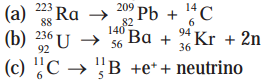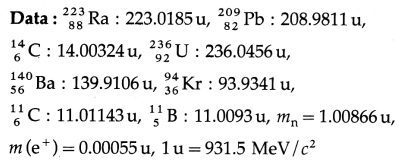(a) $${ }_{88}^{223} \mathrm{Ra} \rightarrow{ }_{82}^{209} \mathrm{~Pb}+{ }_{6}^{14} \mathrm{C}$$
The energy released in this reaction = (∆M) c2
= [223.0185 – (208.9811 + 14.00324)j(931 .5) MeV
= 31.820004 MeV

(b) $${ }_{92}^{236} \mathrm{U} \rightarrow{ }_{56}^{140} \mathrm{Ba}+{ }_{36}^{94} \mathrm{Kr}+2 \mathrm{n}$$
The energy released in this reaction =
(∆M) c2 = [236.0456 – (139.9106 + 93.9341 + (2)(1 .00866)1(93 1 .5)MeV
= 171.00477 MeV

(c) $${ }_{6}^{11} \cdot \mathrm{C} \rightarrow{ }_{5}^{11} \mathrm{~B}+e^{+}$$ + neutrino
The energy released in this reaction = (∆M) c2
= [11.01143 – (11.0093 + O.00055)](931.5) MeV
= 1.47177 MeV

Question 16.
Sample of carbon obtained from any living organism has a decay rate of 15.3 decays per gram per minute. A sample of carbon obtained from very old charcoal shows a disintegration rate of 12.3 disintegrations per gram per minute. Determine the age of the old sample given the decay constant of carbon to be 3.839 × 10-12 per second.
Data: 15.3 decays per gram per minute (living organism), 12.3 disintegrations per gram per minute (very old charcoal). Hence, we have,
$$\frac{A(t)}{A_{0}}=\frac{12.3}{15.3}$$, λ = 3.839 × 10-12 per second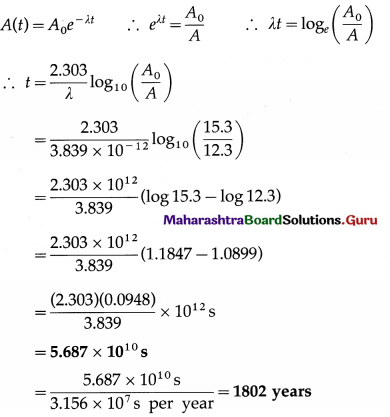Question 17.
The half-life of $${ }_{38}^{90} \mathrm{Sr}$$ is 28 years. Determine the disintegration rate of its 5 mg sample.
Data: T1/2 = 28 years = 28 × 3.156 × 107 s
=8.837 × 108s, M = 5 mg =5 × 10-3g
90 grams of $${ }_{38}^{90} \mathrm{Sr}$$ contain 6.02 × 1023 atoms
Hence, here, N = $$\frac{\left(6.02 \times 10^{23}\right)\left(5 \times 10^{-3}\right)}{90}$$
= 3.344 × 1019 atoms
∴ The disintegration rate = Nλ = N$$\frac{0.693}{T_{1 / 2}}$$
= $$\frac{\left(3.344 \times 10^{19}\right)(0.693)}{8.837 \times 10^{8}}$$
= 2.622 × 1010 disintegrations per secondQuestion 18.
What is the amount of $${ }_{27}^{60} \mathrm{Co}$$ necessary to provide a radioactive source of strength 10.0 mCi, its half-life being 5.3 years?
Data : Activity = 10.0 mCi = 10.0 × 10-3 Ci
= (10.0 × 10-3)(3.7 × 1010) dis/s = 3.7 × 108 dis/s
T1/2 = 5.3 years = (5.3)(3.156 × 107) s
= 1.673 × 108 s
Decay constant, λ = $$\frac{0.693}{T_{1 / 2}}=\frac{0.693}{1.673 \times 10^{8}} \mathrm{~s}^{-1}$$
=4.142 × 10-9 s-1
Activity = Nλ
∴ N = $$\frac{\text { activity }}{\lambda}=\frac{3.7 \times 10^{8}}{4.142 \times 10^{-9}} \text { atoms }$$
= 8.933 × 1016 atoms
=60 grams of $${ }_{27}^{60} \mathrm{Co}$$ contain 6.02 × 1023 atoms
Mass of 8.933 × 1016 atoms of $${ }_{27}^{60} \mathrm{Co}$$
= $$\frac{8.933 \times 10^{16}}{6.02 \times 10^{23}} \times 60 \mathrm{~g}$$
= 8.903 × 10-6 g = 8.903 µg
This is the required amount.

Question 19.
Disintegration rate of a sample is 1010 per hour at 20 hrs from the start. It reduces to 6.3 × 109 per hour after 30 hours. Calculate its half life and the initial number of radioactive atoms in the sample.
Data : A (t1) = 1010 per hour, where t1 = 20 h,
A (t2) = 6.3 × 109 per hour, where t2 = 30 h
A(t) = A0e-λt ∴ A(t1) = A0e-λt1 and A(t2) = Aoe-λt2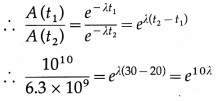∴ 1.587 e10λ ∴ 10λ =2.3031og10(1.587)
∴ λ = (0.2303)(0.2007) = 0.04622 per hour
The half life of the material, T1/2 = $$\frac{0.693}{\lambda}=\frac{0.693}{0.04622}$$
= 14.99 hours
Now, A0 = A (t1)eλt1 = 1010e(0.04622)(20)
= 1010 e0.9244
Let x = e0.9244 ∴ 2.3031og10x = 0.9244
∴ 1og10x = $$\frac{0.9244}{2.303}$$ = 0.4014
∴ x = antilog 0.4014 = 2.52
∴ A0 = 2.52 × 1010 per hour
Now A0 = N0λ ∴ N0 = $$\frac{A_{0}}{\lambda}=\frac{2.52 \times 10^{10}}{0.04622}$$
= 5.452 × 1011
This is the initial number of radioactive atoms in the sample.

Question 20.
The isotope 57Co decays by electron capture to 57Fe with a half-life of 272 d. The 57Fe nucleus is produced in an excited state, and it almost instantaneously emits gamma rays.
(a) Find the mean lifetime and decay constant for 57Co.
(b) If the activity of a radiation source 57Co is 2.0 µCi now, how many 57Co nuclei does the source contain?
(c) What will be the activity after one year?
Data: T1/2 = 272d = 272 × 24 × 60 × 60s = 2.35 × 107 s,
A0 = 2.0uCi = 2.0 × 10-6 × 3.7 × 1010
= 7.4 × 104 dis/s
t = 1 year = 3.156 × 107 s
(a) T1/2 = $$\frac{0.693}{\lambda}$$ = 0.693 τ ∴ The mean lifetime for
57Co = τ = $$\frac{T_{1 / 2}}{0.693}=\frac{2.35 \times 10^{7}}{0.693}$$ = 3391 × 107 s
The decay constant for 57Co = λ = $$\frac{1}{\tau}$$
= $$\frac{1}{3.391 \times 10^{7} \mathrm{~s}}$$
= 2949 × 10-8 s-1

(b)A0 = N0A ∴ N0 = $$\frac{A_{0}}{\lambda}$$ = A0τ
= (7.4 × 104)(3.391 × 107)
= 2.509 × 1012 nuclei
This is the required number.

(c) A(t) = A0e-λt = 2e-(2.949 × 10-8)(3.156 × 107)
= 2e-0.9307 = 2 / e0.9307
Let x = e0.9307 ∴ Iogex = 0.9307
∴ 2.303log10x = 0.9307
∴ log10x = $$\frac{0.9307}{2.303}$$ = 0.4041
∴ x = antilog 0.4041 = 2.536
∴ A (t) = $$\frac{2}{2.536}$$ μCi = 0.7886 μCiQuestion 21.
A source contains two species of phosphorous nuclei, $${ }_{15}^{32} \mathrm{P}$$ (T1/2 = 14.3 d) and $${ }_{15}^{32} \mathrm{P}$$ (T1/2 = 25.3 d). At time t = 0, 90% of the decays are from $${ }_{15}^{32} \mathrm{P}$$ . How much time has to elapse for only 15% of the decays to be from $${ }_{15}^{32} \mathrm{P}$$ ?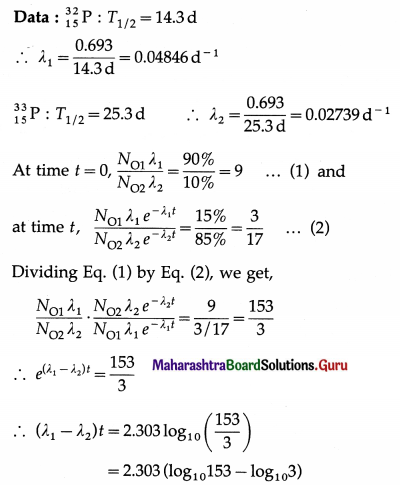∴ (0.04846 – 0.02739) t = 2.303 (2.1847 – 0.4771)
∴ t = $$\frac{(2.303)(1.7076)}{0.02107}$$ = 186.6 days
This is the required time.

Question 22.
Before the year 1900 the activity per unit mass of atmospheric carbon due to the presence of 14C averaged about 0.255 Bq per gram of carbon. (a) What fraction of carbon atoms were 14C? (b) An archaeological specimen containing 500 mg of carbon, shows 174 decays in one hour. What is the age of the specimen, assuming that its activity per unit mass of carbon when the specimen died was equal to the average value of the air? Half-life of 14C is 5730 years?
0.693
Data: T1/2 = 5730y ∴ λ = $$\frac{0.693}{5730 \times 3.156 \times 10^{7}} \mathrm{~s}^{-1}$$
= 3.832 × 10-12 s-1, A = 0.255 Bq per gram of carbon in part (a); M = 500 mg = 500 × 10-3 g,
174 decays in one hour $$\frac{174}{3600}$$ dis/s = 0.04833 dis/s in part (b) (per 500 mg].

(a) A = Nλ ∴ N = $$\frac{A}{\lambda}=\frac{0.255}{3.832 \times 10^{-12}}$$
= 6.654 × 1010
Number of atoms in 1 g of carbon = $$\frac{6.02 \times 10^{23}}{12}$$
=5.017 × 1022
$$\frac{5.017 \times 10^{22}}{6.654 \times 10^{10}}$$ = 0.7539 × 1012
∴ 1 14C atom per 0.7539 × 1012 atoms of carbon
∴ 4 14C atoms per 3 × 1012 atoms of carbon

(b) Present activity per gram = 
= 0.09666 dis/s per gram
A0 = 0.255 dis/s per gram
Now, A(t) = A0e-λt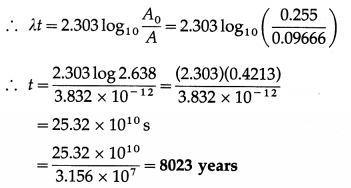This is the required quantity.

Question 23.
How much mass of 235U is required to undergo fission each day to provide 3000 MW of thermal power? Average energy per fission is 202.79 MeV
Data: Power = 3000 MW = 3 × 109 J/s
∴ Energy to be produced each day
=3 × 109 × 86400 J each day
= 2.592 × 1014 J each day
Energy per fission = 202.79 MeV
= 202.79 × 106 × 1.6 × 10-19 J = 3,245 × 10-11 J
∴ Number of fissions each day
= $$\frac{2.592 \times 10^{14}}{3.245 \times 10^{-11}}$$ × 1024 each day
0.235 kg of 235U contains 6.02 × 1023 atoms
7988 x 1024
∴ M = $$\left(\frac{7.988 \times 10^{24}}{6.02 \times 10^{23}}\right)$$ (o.235) = 3.118 kg
This is the required quantity.Question 24.
In a periodic table the average atomic mass of magnesium is given as 24.312 u. The average value is based on their relative natural abundance on earth. The three isotopes and their masses are $${ }_{12}^{24} \mathrm{Mg}$$ (23.98504 u), $${ }_{12}^{25} \mathrm{Mg}$$ (24.98584 u) and $${ }_{12}^{26} \mathrm{Mg}$$ (25.98259 u). The natural abundance of $${ }_{12}^{24} \mathrm{Mg}$$ is 78.99% by mass. Calculate the abundances of other two isotopes.